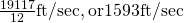5. Integration

# Chapter 5 Review Exercises

True or False. Justify your answer with a proof or a counterexample. Assume all functions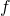and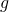are continuous over their domains.

1. If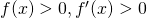for all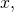then the right-hand rule underestimates the integral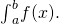Use a graph to justify your answer.

#### Solution

False

2.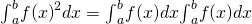3. If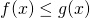for all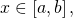then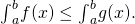#### Solution

True

4. All continuous functions have an antiderivative.

Evaluate the Riemann sums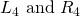for the following functions over the specified interval. Compare your answer with the exact answer, when possible, or use a calculator to determine the answer.

5.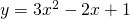over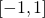#### Solution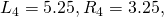exact answer: 4

6.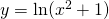over7.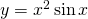over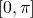#### Solution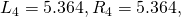exact answer: 5.870

8.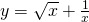over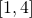Evaluate the following integrals.

9.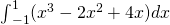#### Solution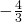10.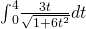11.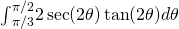#### Solution

1

12.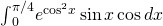Find the antiderivative.

13.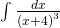#### Solution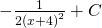14.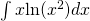15.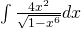#### Solution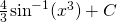16.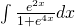Find the derivative.

17.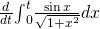#### Solution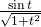18.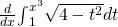19.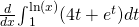#### Solution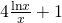20.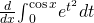The following problems consider the historic average cost per gigabyte of RAM on a computer.

Year 5-Year Change ($) 1980 0 1985 −5,468,750 1990 755,495 1995 −73,005 2000 −29,768 2005 −918 2010 −177 21. If the average cost per gigabyte of RAM in 2010 is$12, find the average cost per gigabyte of RAM in 1980.

#### Solution

$6,328,113 22. The average cost per gigabyte of RAM can be approximated by the function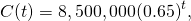whereis measured in years since 1980, and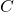is cost in US$. Find the average cost per gigabyte of RAM for 1980 to 2010.

23. Find the average cost of 1GB RAM for 2005 to 2010.

#### Solution

\$73.36

24. The velocity of a bullet from a rifle can be approximated by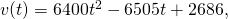whereis seconds after the shot and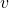is the velocity measured in feet per second. This equation only models the velocity for the first half-second after the shot: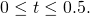What is the total distance the bullet travels in 0.5 sec?

25. What is the average velocity of the bullet for the first half-second?

#### Solution